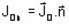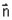# Electric Conduction face (3D) / line (2D) region

## Introduction

The face (in 3D) / line (in 2D) regions are:

• either material regions: used to model thin regions in the study domain

• or non-material regions: used to impose boundary conditions (BC) inside or on the frontiers of the study domain

## Material regions (thin regions)

Thin regions enable the modeling of conducting regions of small thickness (cracks, air-gap, etc.).

The thin regions are described in the same way as the massive regions, with in addition the thickness of the regions.

In 3D, the direction of the E and D fields (and the current) is selected by the user, as indicated in the table below.

Thin region Direction of the electric field (and the current)
no restriction quasi normal quasi tangential
conducting thin region with random ρ resistivity

thin region with: ρ2 >> ρ1thin region with: ρ2 << ρ1## Non-material regions

Non-material regions enable to impose the boundaries conditions (BC).

A region… is a BC that enables one to impose… It is defined by…
Insulating (a potential jump) (predefined)
Imposed current density a current density

a current density (in A/mm²) (formula with input/output parameters or formula with spatial quantities)

Imposed electric potential an electric potential

an electric potential (in V) (formula with input/output parameters or formula with spatial quantities)

Normal electric field a normal electric field
Tangential electric field a tangential electric field
… on the faces/lines that form the region
Warning:whereis the normal component to the region with exterior direction (i.e. having the direction out from the volume region).